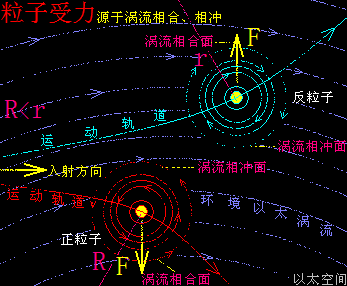# 带电粒子在匀强磁场中运动

1、A+B，与A+B¯。两者结果叠加A+B+A+B¯= 2A=\= 0，表现为宇称不守恒，

1、A¯+B，与A¯+B¯。两者结果叠加A¯+B+A¯+B¯= -2A¯=\= 0，表现为宇称不守恒。

f ∝A+B，或f ∝A+B¯。

f = F（A+B）= Mv^2/R

f¯ = F（A+B¯）= mv^2/rqvB＝Mv^2/R＝mv^2/r